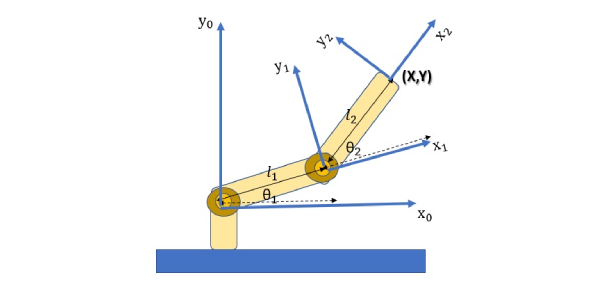Physics Quiz: Kinematics

10 Questions | Total Attempts: 20085SettingsKinematics is the branch of classical mechanics concerned with the motion of various objects without reference to the forces which cause the motion. This physics quiz consists of ten questions of Kinematics to test your knowledge of the topic. If you have been studying it in your physics classes, this quiz can tell you how much you have learned and how much you need to.

• 1.
A person walks due East for 10 meters and then due North for 10 meters. What is the total distance traveled?
• A.

10 meters

• B.

14.14 meters

• C.

20 meters

• D.

20.14 meters

• 2.
A person walks due East for 10 meters and then due North for 10 meters. What is the total displacement?
• A.

10 meters

• B.

14.14 meters

• C.

20 meters

• D.

20.14 meters

• 3.
What is the average speed of a car that travels 30 meters in 10 seconds, then another 50 meters in 30 seconds?
• A.

0.5 m/s

• B.

2 m/s

• C.

20 m/s

• D.

80 m/s

• 4.
What is the velocity of a car that travels -40 meters in 5 seconds?
• A.

45 m/s

• B.

-35 m/s

• C.

8 m/s

• D.

-8 m/s

• 5.
What is the velocity of a car that travels -50 meters in 5 seconds, then 10 meters in 5 seconds?
• A.

-4 m/s

• B.

-10 m/s

• C.

10 m/s

• D.

40 m/s

• 6.
What is the acceleration of a person that goes from 0 m/s to 25 m/s in 5 seconds?
• A.

30 m/s

• B.

20 m/s

• C.

10 m/s

• D.

5 m/s

• 7.
What is the acceleration of a person that goes from 50 m/s to 40 m/s in 10 seconds?
• A.

-1 m/s

• B.

1 m/s

• C.

-9 m/s

• D.

9 m/s

• 8.
What is the average velocity during the first 10 seconds?
• A.

60 m/s

• B.

30 m/s

• C.

10 m/s

• D.

6 m/s

• 9.
What is the average velocity from 20 to 30 seconds?
• A.

-40 m/s

• B.

-30 m/s

• C.

-4 m/s

• D.

10 m/s

• 10.
Where is the velocity equal to 0 m/s?
• A.

5 s

• B.

12 s

• C.

30 s

• D.

54 s

Related TopicsBack to top